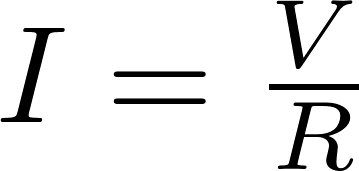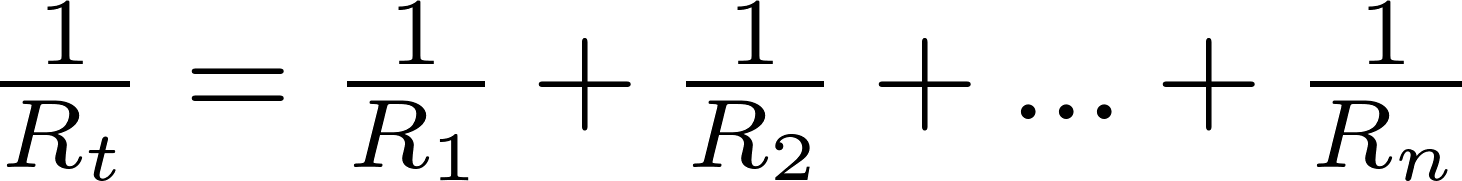# Resistor

The resistor in a passive electrical component that can be found in nearly all electronic devices. Resistors have a resistance that reduces the current flow through a circuit. The resistance is measured in ohms (Ω).

### Ohm's law

Ohm's law

The behavior of the resistor is described by Ohm's law. Ohm's law states that the current is equal to the voltage divided by the resistance:This formula shows that the current will decrease when the resistance increases. If, for example, we have a 20 ohm resistor connected to a 5 volt battery, there will flow a current of 5/20 = 0.25 amperes through the resistor.

### Resistors in series

Resistors in series

Resistors can be connected in series. The resistance is equal to the sum of all individual resistances.### Resistors in parallel

Resistors in parallel

Resistors can also be connected in parallel. The resistance in this situation will be equal to the reciprocal (inverse) of the sum of the reciprocal of all individual resistances.### Markings

#### Color code

The resistance of most resistors can be calculated by the colored bands. Four band resistors are the most common. The first two bands represent a number between 00 and 99. This number is multiplied by the value represented by the third band. The last band gives the tolerance. You can find more information about the color codes here.

color digit multiplication tolerance
black 0 1 -
brown 1 10 1%
red 2 100 2%
orange 3 1000 3%
yellow 4 10,000 4%
green 5 100,000 0.5%
blue 6 1,000,000 0.25%
violet 7 10,000,000 0.1%
grey 8 100,000,000 0.05%
white 9 1,000,000,000 -
gold - 0.1 5%
silver - 0.01 10%

#### SMD code

SMD resistors use numbers and letters instead of colors. Most SMD resistors have three numbers. The resistance is equal to the first two digits multiplied by ten to the power of the third digit. You can find more information about SMD codes here